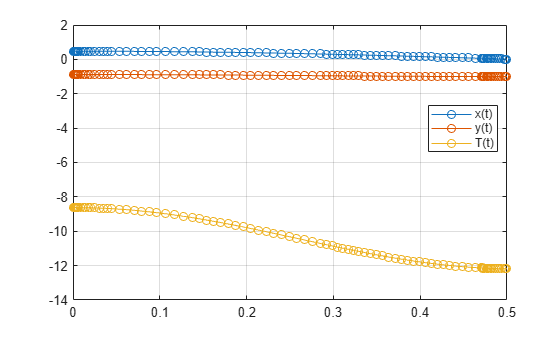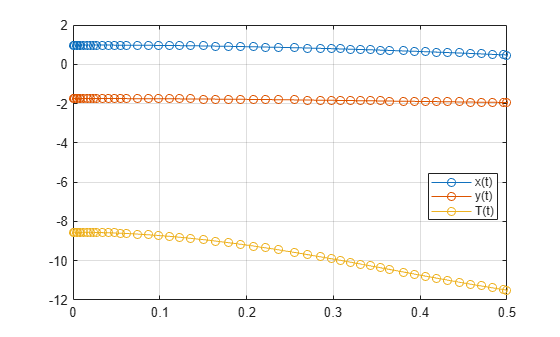## Solve DAEs Using Mass Matrix Solvers

Solve differential algebraic equations by using one of the mass matrix solvers available in MATLAB®. To use this workflow, first complete steps 1, 2, and 3 from Solve Differential Algebraic Equations (DAEs). Then, use a mass matrix solver instead of `ode15i`.

This example demonstrates the use of `ode15s` or `ode23t`. For details on the other solvers, see Choose an ODE Solver (MATLAB) and adapt the workflow on this page.

### Step 1. Convert DAEs to Function Handles

From the output of `reduceDAEIndex`, you have a vector of equations `DAEs` and a vector of variables `DAEvars`. To use `ode15s` or `ode23t`, you need two function handles: one representing the mass matrix of a DAE system, and the other representing the right sides of the mass matrix equations. These function handles form the equivalent mass matrix representation of the ODE system where M(t,y(t))y’(t) = f(t,y(t)).

Find these function handles by using `massMatrixForm` to get the mass matrix `M` and the right sides `F`.

`[M,f] = massMatrixForm(DAEs,DAEvars)`
```M = [ 0, 0, 0, 0, 0, 0, 0] [ 0, 0, 0, 0, 0, 0, 0] [ 0, 0, 0, 0, 0, 0, 0] [ 0, 0, 0, 0, 0, 0, 0] [ 0, 0, 0, 0, 0, 0, 0] [ 0, 0, 0, 0, -1, 0, 0] [ 0, -1, 0, 0, 0, 0, 0] f = (T(t)*x(t) - m*r*Dxtt(t))/r -(g*m*r - T(t)*y(t) + m*r*Dytt(t))/r r^2 - y(t)^2 - x(t)^2 - 2*Dxt(t)*x(t) - 2*Dyt(t)*y(t) - 2*Dxtt(t)*x(t) - 2*Dytt(t)*y(t) - 2*Dxt(t)^2 - 2*Dyt(t)^2 -Dytt(t) -Dyt(t)```

The equations in `DAEs` can contain symbolic parameters that are not specified in the vector of variables `DAEvars`. Find these parameters by using `setdiff` on the output of `symvar` from `DAEs` and `DAEvars`.

```pDAEs = symvar(DAEs); pDAEvars = symvar(DAEvars); extraParams = setdiff(pDAEs, pDAEvars)```
```extraParams = [ g, m, r]```

The mass matrix `M` does not have these extra parameters. Therefore, convert `M` directly to a function handle by using `odeFunction`.

`M = odeFunction(M, DAEvars);`

Convert `f` to a function handle. Specify the extra parameters as additional inputs to `odeFunction`.

`f = odeFunction(f, DAEvars, g, m, r);`

The rest of the workflow is purely numerical. Set parameter values and create the function handle.

```g = 9.81; m = 1; r = 1; F = @(t, Y) f(t, Y, g, m, r);```

### Step 2. Find Initial Conditions

The solvers require initial values for all variables in the function handle. Find initial values that satisfy the equations by using the MATLAB `decic` function. The `decic` accepts guesses (which might not satisfy the equations) for the initial conditions, and tries to find satisfactory initial conditions using those guesses. `decic` can fail, in which case you must manually supply consistent initial values for your problem.

First, check the variables in `DAEvars`.

`DAEvars`
```DAEvars = x(t) y(t) T(t) Dxt(t) Dyt(t) Dytt(t) Dxtt(t)```

Here, `Dxt(t)` is the first derivative of `x(t)`, `Dytt(t)` is the second derivative of `y(t)`, and so on. There are 7 variables in a `7`-by-`1` vector. Thus, guesses for initial values of variables and their derivatives must also be `7`-by-`1` vectors.

Assume the initial angular displacement of the pendulum is 30° or `pi/6`, and the origin of the coordinates is at the suspension point of the pendulum. Given that we used a radius `r` of `1`, the initial horizontal position `x(t)` is `r*sin(pi/6)`. The initial vertical position `y(t)` is `-r*cos(pi/6)`. Specify these initial values of the variables in the vector `y0est`.

Arbitrarily set the initial values of the remaining variables and their derivatives to `0`. These are not good guesses. However, they suffice for our problem. In your problem, if `decic` errors, then provide better guesses and refer to the `decic` page.

```y0est = [r*sin(pi/6); -r*cos(pi/6); 0; 0; 0; 0; 0]; yp0est = zeros(7,1);```

Create an option set that contains the mass matrix `M` and initial guesses `yp0est`, and specifies numerical tolerances for the numerical search.

```opt = odeset('Mass', M, 'InitialSlope', yp0est,... 'RelTol', 10.0^(-7), 'AbsTol' , 10.0^(-7));```

Find consistent initial values for the variables and their derivatives by using the MATLAB `decic` function. The first argument of `decic` must be a function handle describing the DAE as `f(t,y,yp) = f(t,y,y') = 0`. In terms of `M` and `F`, this means ```f(t,y,yp) = M(t,y)*yp - F(t,y)```.

```implicitDAE = @(t,y,yp) M(t,y)*yp - F(t,y); [y0, yp0] = decic(implicitDAE, 0, y0est, [], yp0est, [], opt)```
```y0 = 0.4771 -0.8788 -8.6214 0 0.0000 -2.2333 -4.1135 yp0 = 0 0.0000 0 0 -2.2333 0 0```

Now create an option set that contains the mass matrix `M` of the system and the vector `yp0` of consistent initial values for the derivatives. You will use this option set when solving the system.

`opt = odeset(opt, 'InitialSlope', yp0);`

### Step 3. Solve DAE System

Solve the system integrating over the time span `0``t``0.5`. Add the grid lines and the legend to the plot. The code uses `ode15s`. Instead, you can use `ode23s` by replacing `ode15s` with `ode23s`.

```[tSol,ySol] = ode15s(F, [0, 0.5], y0, opt); plot(tSol,ySol(:,1:origVars),'-o') for k = 1:origVars S{k} = char(DAEvars(k)); end legend(S, 'Location', 'Best') grid on```Solve the system for different parameter values by setting the new value and regenerating the function handle and initial conditions.

Set `r` to `2` and regenerate the function handle and initial conditions.

```r = 2; F = @(t, Y) f(t, Y, g, m, r); y0est = [r*sin(pi/6); -r*cos(pi/6); 0; 0; 0; 0; 0]; implicitDAE = @(t,y,yp) M(t,y)*yp - F(t,y); [y0, yp0] = decic(implicitDAE, 0, y0est, [], yp0est, [], opt); opt = odeset(opt, 'InitialSlope', yp0);```

Solve the system for the new parameter value.

```[tSol,ySol] = ode15s(F, [0, 0.5], y0, opt); plot(tSol,ySol(:,1:origVars),'-o') for k = 1:origVars S{k} = char(DAEvars(k)); end legend(S, 'Location', 'Best') grid on```## Related Topics

#### Mathematical Modeling with Symbolic Math Toolbox

Get examples and videos